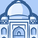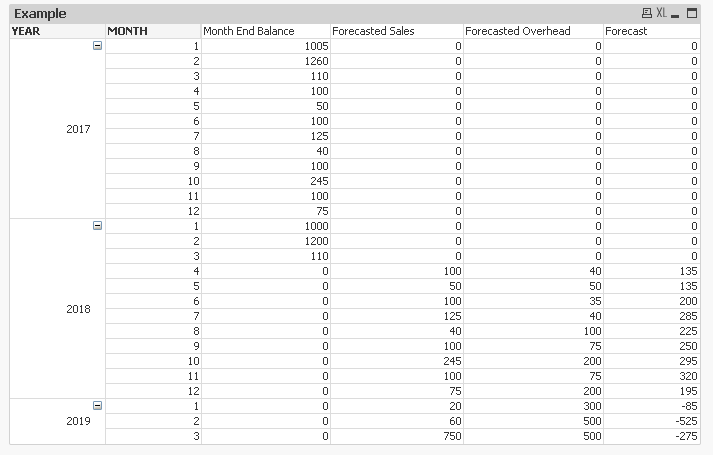# QlikView App Dev

Discussion Board for collaboration related to QlikView App Development.

Announcements
cancel
Showing results for
Did you mean:Contributor III

## Forecasting future months data using data in different dimensions

Hello Qlik community,

Summary

I am attempting to project month end balance amounts for the remainder of the year by utilizing forecast data from 2 different dimensions as well as current monthend balance amounts for the current month.

Method

Honestly, the math is pretty simple however the tricky part is I'm out of ideas as how I can forecast past the current month. I have attached an example to this topic. Basically I need to take the "month end balance" for March, add April's forecast income and then subtract April's forecast expenses to determine the forecast month end balance for April.

Once this is done, I need to do the same math for the remainder of the year, however, each for month after April, I will need to use the prior month's forecast month end balance that I am calculating.

The excel spreadsheet I attached shows the desired results of what I'm attempting to do and I have a qvw file with the same data.

Current Solution

In reality, I have created a stored SQL procedure that runs every month to give forecast results. Then I pull the data into Qlikview. This works however it does limit some of the development/design I would like to do and is quite cumbersome to maintain in SQL if any updates arise from the business. Ultimately being able to do this in a Qlikview edit script or a Qlikview expression would be ideal. The major issue I'm having is I'm hitting a brick wall as to how I can tell Qlikview to take the prior month's forecast amount which I have calculated and then add and subtract the forecast sales and expenses which is data that is present/not calculated.

Any help on this topic would be strongly appreciated as I can not seem to find a solution. If anything was confusing or if more context is needed I'll be happy to explain further.

Thanks,

-Brandon-

8 RepliesMVP

Try this

=If(Sum(ENDING_AMOUNT) = 0, RangeSum(Sum(TOTAL {<MONTH = {'\$(=Max({<MONTH = {"=Sum(ENDING_AMOUNT) <> 0"}>} MONTH))'}>} ENDING_AMOUNT), RangeSum(Above(Sum(SALES), 0, RowNo())), -RangeSum(Above(Sum(OVERHEAD), 0, RowNo()))), 0)Contributor III
Author

Sunny,

Thanks for your assistance the expression you provided worked as expected. Now I just have to figure out how to handle a data set where there are multiple Years included.Contributor III
Author

Hi Sunny,

Sorry to bother you again but how would you suggest rewriting the expression to compensate for a table with more than one year's worth of data? The "Max Month" will be 12 since the previous year has values for each month. I have attached an example.MVP

Not sure I understand, but are you looking for this?=If(Sum(ENDING_AMOUNT) = 0, RangeSum(Sum(TOTAL {<MONTH = {'\$(=Max({<MONTH = {"=Sum(ENDING_AMOUNT) <> 0"}>} MONTH))'}>} ENDING_AMOUNT), RangeSum(Above(TOTAL Sum(SALES), 0, RowNo(TOTAL))), -RangeSum(Above(TOTAL Sum(OVERHEAD), 0, RowNo(TOTAL)))), 0)Contributor III
Author

Sorry for the confusion. I tried that solution and got the same numbers however the values are incorrect in the forecast column.

Using the current expression, the expression is taking the "Month End Balance" from December 2017, adding the Forecasted Sales for April 2018 and subtracting the Forecasted Overhead for April 2018. So the Math would be 75+100-40=135.

Instead, the expression should take the "Month End Balance" from March 2018, add the Forecasted Sales for April 2018 and subtract the Forecasted Overhead for April 2018. So the Math would be 110+100-40=170.

170 should be the correct value for "Forecast" April 2018. The {<MONTH = {'\$(=Max({<MONTH = {"=Sum(ENDING_AMOUNT) <> 0"}>} MONTH))'}>} part of the expression is taking the max month in the data set which is 12. I think this is whats causing the expression to not calculate the desired results. Is there any way to tell the expression to take the Max Month of the Current Year "2018"?MVP

=If(Sum(ENDING_AMOUNT) = 0, RangeSum(Sum(TOTAL {<MONTH_YEAR = {'\$(=Max({<MONTH_YEAR = {"=Sum(ENDING_AMOUNT) <> 0"}>} MONTH_YEAR))'}>} ENDING_AMOUNT), RangeSum(Above(TOTAL Sum(SALES), 0, RowNo(TOTAL))), -RangeSum(Above(TOTAL Sum(OVERHEAD), 0, RowNo(TOTAL)))), 0)

Here MONTH_YEAR is created in script like this

TEST_SALES:

Num(MakeDate(YEAR, MONTH)) as MONTH_YEAR;

[YEAR, MONTH, ENDING_AMOUNT, SALES, OVERHEAD, FCST_AMOUNT

2017, 1, 1005, 0, 0,

2017, 2, 1260, 0, 0,

2017, 3, 110, 0, 0,

2017, 4, 100, 0, 0,

2017, 5, 50, 0, 0,

2017, 6, 100, 0, 0,

2017, 7, 125, 0, 0,Contributor III
Author

Sunny,

Sorry for the delay in response. Your solution worked perfectly with what I was attempting to accomplish.

Thank youMVP

That is great!! I glad it all worked out well for you